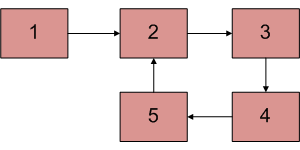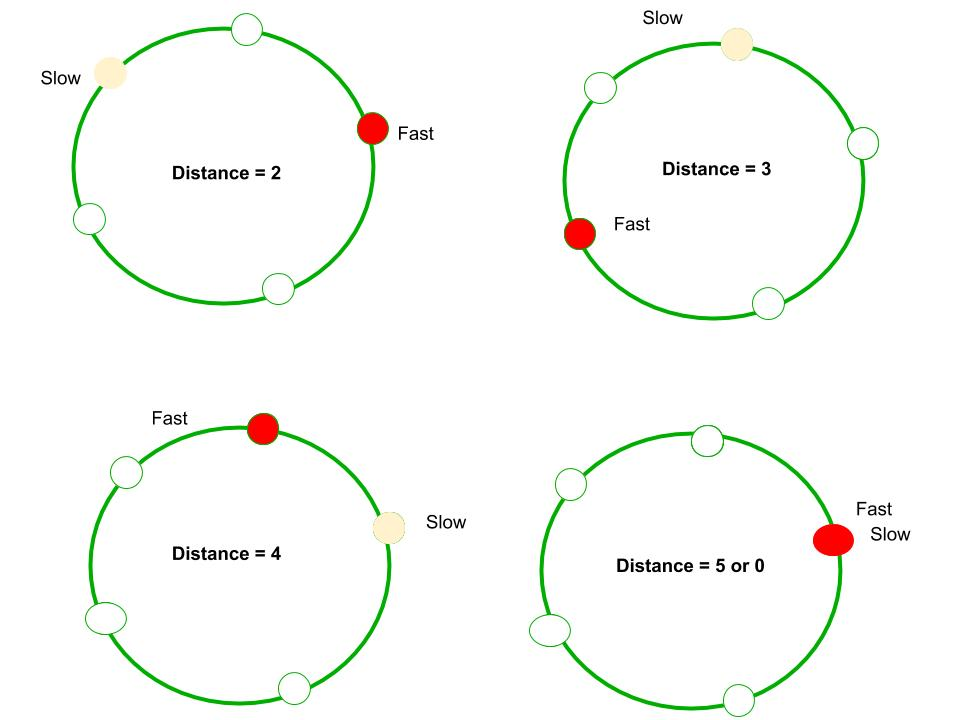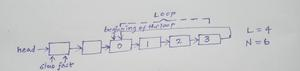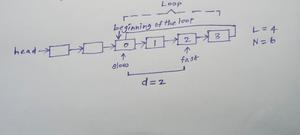Open in App
Not now

# How does Floyd’s slow and fast pointers approach work?

• Difficulty Level : Easy
• Last Updated : 11 Jan, 2023

We have discussed Floyd’s fast and slow pointer algorithms in Detect loop in a linked listThe algorithm is to start two pointers, slow and fast from head of linked list. We move slow one node at a time and fast two nodes at a time. If there is a loop, then they will definitely meet. This approach works because of the following facts. 1) When slow pointer enters the loop, the fast pointer must be inside the loop.

Let fast pointer be distance k from slow. 2) Now if consider movements of slow and fast pointers, we can notice that distance between them (from slow to fast) increase by one after every iteration. After one iteration (of slow = next of slow and fast = next of next of fast), distance between slow and fast becomes k+1, after two iterations, k+2, and so on. When distance becomes n, they meet because they are moving in a cycle of length n. For example, we can see in below diagram, initial distance is 2. After one iteration, distance becomes 3, after 2 iterations, it becomes 4. After 3 iterations, it becomes 5 which is distance 0. And they meet.Alternate Proof:

• Let us give index to all the nodes of the loop starting from 0Index is assigned to each node of the loop

• Let the length of the Loop be L
• Let us observe the movement of the Slow pointer from the beginning of the loop along with iterations.

From the table , we can say that

Index of the node pointed by Slow pointer after iteration i = i mod L

• Let us observe the movement of Fast pointer from the beginning of the loop along with iterations.

From the table, we can say that

Index of the node pointed by Fast pointer after iteration i = ( 2 x i ) mod L

• One thing to note is that , by the time slow pointer reaches the beginning of the loop , the fast pointer will be d nodes away from the beginning of the loop
• 0 <= d <= L-1By the time slow pointer reaches the beginning of the loop, fast pointer is d nodes away from the beginning of the loop

• Hence for all the consequent iterations too , the Fast pointer will be d nodes away from the node that it would point if it started from beginning of the loop
• Hence we have
Index of the node pointed by Fast pointer after iteration i = ( ( 2 x i ) + d ) mod L

Now we have both the equations :

• Index of the node pointed by Slow pointer after iteration i = i mod L
• Index of the node pointed by Fast pointer after iteration i = ( ( 2 x i ) + d ) mod L

Now assign i = c , where c = L – d

L – Length of the loop

d – distance between the Fast pointer and beginning of the loop , when Slow pointer reaches the beginning of the loop

Since 0 <= d <= L-1 , implies  1 <= c <= L

• Index of the node pointed by Slow pointer after iteration i  = i mod L
= c mod L
= 0 , ( if c = L )
c , ( if c < L )
• Index of the node pointed by Fast pointer after iteration i   = ( ( 2 x i ) + d ) mod L
= ( ( 2 x c ) + d ) mod L
= ( 2c + d ) mod L
= ( c + c + d ) mod L
= ( c + L ) mod L  , Since c = L – d , implies c + d = L
= c mod L
= 0 , ( if c = L )
c , ( if c < L )
• Now Both the Slow pointer and the Fast pointer are pointing to the same node
• Hence proved

How does cycle removal algorithm work? Please see method 3 of Detect and Remove Loop in a Linked List

Implementation:

## Java

 `// Java program to detect loop in a linked list``class` `LinkedList {``    ``Node head; ``// head of list`` ` `    ``/* Linked list Node*/``    ``class` `Node {``        ``int` `data;``        ``Node next;``        ``Node(``int` `d)``        ``{``            ``data = d;``            ``next = ``null``;``        ``}``    ``}`` ` `    ``/* Inserts a new Node at front of the list. */``    ``public` `void` `push(``int` `new_data)``    ``{``        ``/* 1 & 2: Allocate the Node &``                ``Put in the data*/``        ``Node new_node = ``new` `Node(new_data);`` ` `        ``/* 3. Make next of new Node as head */``        ``new_node.next = head;`` ` `        ``/* 4. Move the head to point to new Node */``        ``head = new_node;``    ``}`` ` `    ``void` `detectLoop()``    ``{``        ``Node slow_p = head, fast_p = head;``        ``int` `flag = ``0``;``        ``while` `(slow_p != ``null` `&& fast_p != ``null``            ``&& fast_p.next != ``null``) {``            ``slow_p = slow_p.next;``            ``fast_p = fast_p.next.next;``            ``if` `(slow_p == fast_p) {``                ``flag = ``1``;``                ``break``;``            ``}``        ``}``        ``if` `(flag == ``1``)``            ``System.out.println(``"Loop found"``);``        ``else``            ``System.out.println(``"Loop not found"``);``    ``}`` ` `    ``/* Driver program to test above functions */``    ``public` `static` `void` `main(String args[])``    ``{``        ``LinkedList llist = ``new` `LinkedList();`` ` `        ``llist.push(``20``);``        ``llist.push(``4``);``        ``llist.push(``15``);``        ``llist.push(``10``);`` ` `        ``/*Create loop for testing */``        ``llist.head.next.next.next.next = llist.head;`` ` `        ``llist.detectLoop();``    ``}``}`

## C++

 `// C++ program to detect loop in a linked list``#include ``using` `namespace` `std;`` ` `/* Link list node */``class` `Node {``public``:``    ``int` `data;``    ``Node* next;``};`` ` `void` `push(Node** head_ref, ``int` `new_data)``{``    ``/* allocate node */``    ``Node* new_node = ``new` `Node();`` ` `    ``/* put in the data */``    ``new_node->data = new_data;`` ` `    ``/* link the old list of the new node */``    ``new_node->next = (*head_ref);`` ` `    ``/* move the head to point to the new node */``    ``(*head_ref) = new_node;``}`` ` `int` `detectLoop(Node* list)``{``    ``Node *slow_p = list, *fast_p = list;`` ` `    ``while` `(slow_p && fast_p && fast_p->next) {``        ``slow_p = slow_p->next;``        ``fast_p = fast_p->next->next;``        ``if` `(slow_p == fast_p) {``            ``return` `1;``        ``}``    ``}``    ``return` `0;``}`` ` `/* Driver code*/``int` `main()``{``    ``/* Start with the empty list */``    ``Node* head = NULL;`` ` `    ``push(&head, 20);``    ``push(&head, 4);``    ``push(&head, 15);``    ``push(&head, 10);`` ` `    ``/* Create a loop for testing */``    ``head->next->next->next->next = head;``    ``if` `(detectLoop(head))``        ``cout << ``"Loop found"``;``    ``else``        ``cout << ``"No Loop"``;``    ``return` `0;``}`

## Javascript

 ``

## Python

 `# Python program to detect loop in the linked list`` ` `# Node class`` ` ` ` `class` `Node:`` ` `    ``# Constructor to initialize the node object``    ``def` `__init__(``self``, data):``        ``self``.data ``=` `data``        ``self``.``next` `=` `None`` ` ` ` `class` `LinkedList:`` ` `    ``# Function to initialize head``    ``def` `__init__(``self``):``        ``self``.head ``=` `None`` ` `    ``# Function to insert a new node at the beginning``    ``def` `push(``self``, new_data):``        ``new_node ``=` `Node(new_data)``        ``new_node.``next` `=` `self``.head``        ``self``.head ``=` `new_node`` ` `    ``# Utility function to print it the linked LinkedList``    ``def` `printList(``self``):``        ``temp ``=` `self``.head``        ``while``(temp):``            ``print` `temp.data,``            ``temp ``=` `temp.``next`` ` `    ``def` `detectLoop(``self``):``        ``slow_p ``=` `self``.head``        ``fast_p ``=` `self``.head``        ``while``(slow_p ``and` `fast_p ``and` `fast_p.``next``):``            ``slow_p ``=` `slow_p.``next``            ``fast_p ``=` `fast_p.``next``.``next``            ``if` `slow_p ``=``=` `fast_p:``                ``return`` ` ` ` `# Driver program for testing``llist ``=` `LinkedList()``llist.push(``20``)``llist.push(``4``)``llist.push(``15``)``llist.push(``10``)`` ` `# Create a loop for testing``llist.head.``next``.``next``.``next``.``next` `=` `llist.head``if``(llist.detectLoop()):``    ``print` `"Found Loop"``else``:``    ``print` `"No Loop"`

## C#

 `// C# program to detect loop in a linked list``using` `System;`` ` `public` `class` `LinkedList {``    ``Node head; ``// head of list`` ` `    ``/* Linked list Node*/``    ``public` `class` `Node {``        ``public` `int` `data;``        ``public` `Node next;``        ``public` `Node(``int` `d)``        ``{``            ``data = d;``            ``next = ``null``;``        ``}``    ``}`` ` `    ``/* Inserts a new Node at front of the list. */``    ``public` `void` `push(``int` `new_data)``    ``{``        ``/* 1 & 2: Allocate the Node &``                ``Put in the data*/``        ``Node new_node = ``new` `Node(new_data);`` ` `        ``/* 3. Make next of new Node as head */``        ``new_node.next = head;`` ` `        ``/* 4. Move the head to point to new Node */``        ``head = new_node;``    ``}`` ` `    ``Boolean detectLoop()``    ``{``        ``Node slow_p = head, fast_p = head;``        ``while` `(slow_p != ``null` `&& fast_p != ``null``            ``&& fast_p.next != ``null``) {``            ``slow_p = slow_p.next;``            ``fast_p = fast_p.next.next;``            ``if` `(slow_p == fast_p) {``                ``return` `true``;``            ``}``        ``}``        ``return` `false``;``    ``}`` ` `    ``/* Driver code */``    ``public` `static` `void` `Main(String[] args)``    ``{``        ``LinkedList llist = ``new` `LinkedList();`` ` `        ``llist.push(20);``        ``llist.push(4);``        ``llist.push(15);``        ``llist.push(10);``        ``/*Create loop for testing */``        ``llist.head.next.next.next.next = llist.head;`` ` `        ``Boolean found = llist.detectLoop();``        ``if` `(found) {``            ``Console.WriteLine(``"Loop Found"``);``        ``}``        ``else` `{``            ``Console.WriteLine(``"No Loop"``);``        ``}``    ``}``}`

Output

`Loop found`

My Personal Notes arrow_drop_up